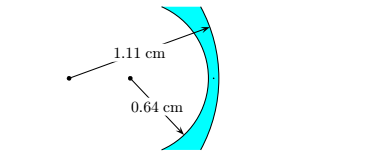Problem: The cross-section of a glass lens with an index of refraction 1.5, is shown below. Determine the focal length f using the small angle approximation. Include both the magnitude and sign, which indicates whether this is a divergent or convergent lens. 1. 0.514286 2. 1.03196 3. -0.902762 4. -3.99724 5. -3.02298 6. 0.992568 7. -3.60345 8. 0.904751 9. 1.08561 10. -0.703276

FREE Expert Solution
91% (263 ratings)
Problem Details

The cross-section of a glass lens with an index of refraction 1.5, is shown below. Determine the focal length f using the small angle approximation. Include both the magnitude and sign, which indicates whether this is a divergent or convergent lens.

1. 0.514286

2. 1.03196

3. -0.902762

4. -3.99724

5. -3.02298

6. 0.992568

7. -3.60345

8. 0.904751

9. 1.08561

10. -0.703276